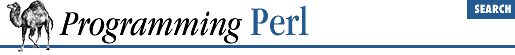#Chapter 3Functions### 3.2.34 exists

`exists `EXPR``

This function returns true if the specified hash key exists in its hash, even if the corresponding value is undefined.

```print "Exists\n" if exists \$hash{\$key};
print "Defined\n" if defined \$hash{\$key};
print "True\n" if \$hash{\$key};```

A hash element can only be true if it's defined, and can only be defined if it exists, but the reverse doesn't necessarily hold true in either case.

`EXPR` can be arbitrarily complicated as long as the final operation is a hash key lookup:

`if (exists \$ref->[\$x][\$y]{\$key}) { ... }`